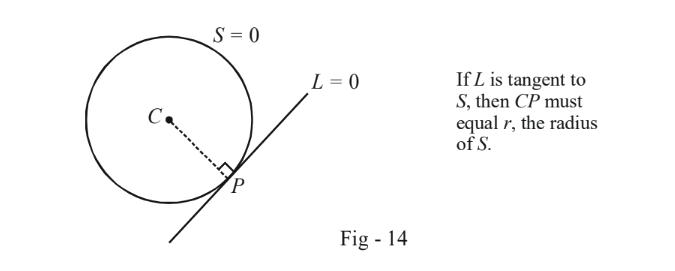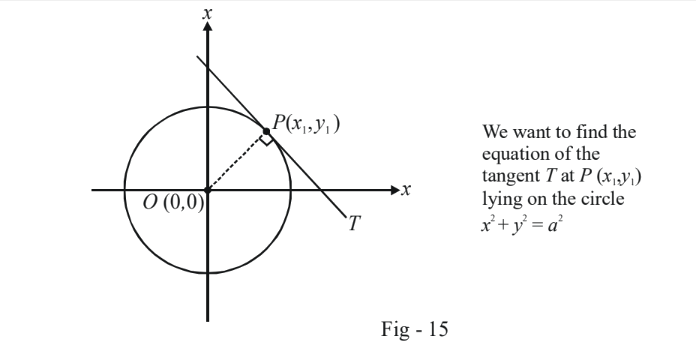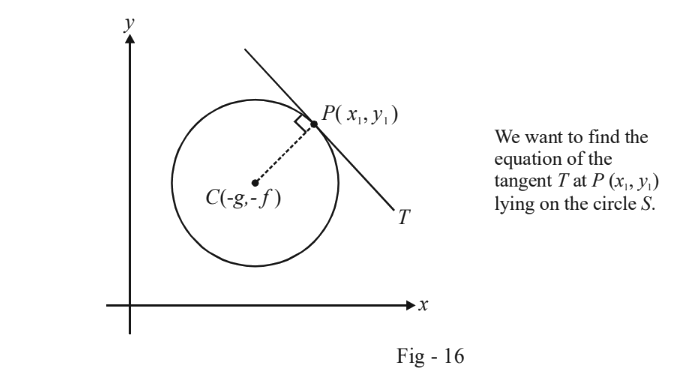# Tangents To Circles

Go back to  'Circles'

We first consider the case which we’ve already considered in Example - 8 earlier, namely, when will the line $$y = mx + c$$ be a tangent to the circle $${x^2} + {y^2} = {a^2}?$$

Earlier, we solved the simultaneous system of two equations and put the discriminant of the resulting quadratic equal to 0 to obtain the condition for tangency. Here, we follow an alternative approach.

We use the simple geometric fact that if a line L is a tangent to a circle S, then the perpendicular distance of the centre of S from L must equal the radius of S:In the current case, the centre of $${x^2} + {y^2} = {a^2}$$ is (0, 0) and its radius is a. The perpendicular distance of (0, 0) from $$y = mx + c$$ must equal a, i.e.

\begin{align}&\frac{{\left| c \right|}}{{\sqrt {1 + {m^2}} }} = a \\\\ \Rightarrow \quad &\fbox{{{c^2} = {a^2}(1 + {m^2})}}\end{align}

This is the same condition that we derived earlier. From this relation, we can infer that any line of the form $$y = mx \pm a\sqrt {1 + {m^2}}$$ will always be a tangent to the circle $${x^2} + {y^2} = {a^2},$$ whatever the value of m may be.

Consider now the problem of finding the equation of the tangent to the circle $${x^2} + {y^2} = {a^2}$$ at a point $$P({x_1},{y_1})$$ lying on the circle.The tangent T will obviously be perpendicular to OP. Thus, the equation of T is

\begin{align}{}\,\,\,\,\,\,\,\,\,\,\,\,\,\,\,\,\,\,\,&\frac{{y - {y_1}}}{{x - {x_1}}} = \frac{{ - {x_1}}}{{{y_1}}}\\\\ \Rightarrow \quad & x{x_1} + y{y_1} = x_1^2 + y_1^2\,\,\,\,\,\,\,\,\,\,\,\,\,\,\,\,\,\,\,...(1)\end{align}

The other piece of information that we have is that $$({x_1},{y_1})$$ lies on the circle and therefore must satisfy its equation. Thus, $$x_1^2 + y_1^2 = {a^2}.$$ Using this in (1), we obtain the equation of T as

$\fbox{\begin{array}{*{20}{c}} {T:\,\,x{x_1} + y{y_1} = {a^2}}\end{array}}$

If the point $$({x_1},{y_1})$$ has been specified in polar form, i.e. in the form $$(a\cos \theta ,a\sin \theta ),$$ the equation of T becomes

$\fbox{\begin{array}{*{20}{c}} {T:\,\,x\cos \theta + y\sin \theta = a}\end{array}}$

We now go to the general case of finding the equation of the tangent to an arbitrary circle $$S \equiv {x^2} + {y^2} + 2gx + 2fy + c = 0$$ at a point $$P({x_1}{y_1})$$ lying on this circle:Here again, as in the earlier case, we have two pieces of information which we can put to use :

\begin{align} \text{ P lies on S} : \qquad \quad &x_1^2 + y_1^2 + 2g{x_1} + 2f{y_1} + c = 0 \qquad\qquad ...(1)\\\\\text{ T is perpendicular to CP} : &\frac{{y - {y_1}}}{{x - {x_1}}} \times \frac{{{y_1} + f}}{{{x_1} + g}} = - 1\,\qquad\left\{ {(x,y){\text{ is any point on }}T} \right\}\\\\ \Rightarrow \qquad &x{x_1} + y{y_1} + gx + fy = x_1^2 + y_1^2 + g{x_1} + f{y_1}\end{align}

We now add $$(g{x_1} + f{y_1} + c)$$ on both sides above :

$$\Rightarrow \quad x{x_1} + y{y_1} + g(x + {x_1}) + f(y + {y_1}) + c = x_1^2 + y_1^2 + 2g{x_1} + 2f{y_1} + c$$

Now we use (1) for the RHS  above to finally obtain the equation of T as

$$\qquad\qquad\qquad \fbox{\begin{array}{*{20}{c}} {T:\,\,x{x_1} + y{y_1} + g(x + {x_1}) + f(y + {y_1}) + c = 0}\end{array}}$$

The expression on the left hand side of the equation above is conventionally denoted as $$T({x_1},{y_1})$$  (Note that T is a function of x and y too). Thus, the equation of the tangent can be written concisely as

$T({x_1},{y_1}) = 0 \quad : \quad \text {Equation of tangent at}\;\;({x_1},{y_1}) \; \; \text{to} \;\;S$

Using an analogous approach, we can write the equation of the normal to the circle $${x^2} + {y^2} + 2gx + 2fy + c = 0$$ at the point $$P\left( {{x_1},{y_1}} \right)$$. You are urged to do this yourself. Note that every normal of a circle will  pass through the circle’s centre.

Example - 16

Find the equation of the tangent to

(a)  $${x^2} + {y^2} = 1$$ at \begin{align}\left( {\frac{1}{{\sqrt 3 }},\frac{{\sqrt 2 }}{{\sqrt 3 }}} \right)\end{align}

(b) $${x^2} + {y^2} - 2x - 4y + 4 = 0$$  at \begin{align}\left( {\frac{1}{2},2 + \frac{{\sqrt 3 }}{2}} \right)\end{align}

Solution (a) The equation of the tangent to $${x^2} + {y^2} = {a^2}$$ at $$({x_1},{y_1})$$ is, as obtained in the preceding discussion,

$\begin{array}{l}\,\,\,\,\,\,\,\,\,\,\,\,\,\,\,\,\,\,&T({x_1},{y_1}) = 0\\\\ \Rightarrow & x{x_1} + y{y_1} = {a^2}\end{array}$

Here, \begin{align}({x_1},{y_1}) = \left( {\frac{1}{{\sqrt 3 }},\frac{{\sqrt 2 }}{{\sqrt 3 }}} \right)\end{align} and $$a = 1$$ . Thus, the required equation is $$x + \sqrt 2 y = \sqrt 3$$

(b) The equation of the tangent to $${x^2} + {y^2} + 2gx + 2fy + c = 0$$  at $$({x_1},{y_1})$$ is

$\begin{array}{l} \,\,\,\,\,\,\,\,\,\,\,\,\,\,\,\,&T({x_1},{y_1}) = 0\\\\ \Rightarrow & x{x_1} + y{y_1} + g(x + {x_1}) + f(y + {y_1}) + c = 0 \end{array}$

Here, $$({x_1},{y_1})$$ is \begin{align}\left( {\frac{1}{2},2 + \frac{{\sqrt 3 }}{2}} \right)\end{align} and thus, the required equation is

\begin{align}{}\,\,\,\,\,\,\,\,\,\,\,\,\,\,\,\,\,&\frac{x}{2} + \left( {2 + \frac{{\sqrt 3 }}{2}} \right)y - 1\left( {x + \frac{1}{2}} \right) - 2\left( {y + 2 + \frac{{\sqrt 3 }}{2}} \right) + 4 = 0\\\\\Rightarrow \qquad & \frac{{ - x}}{2} + \frac{{\sqrt 3 y}}{2} - \left( {\sqrt 3 + \frac{1}{2}} \right) = 0\\\\ \Rightarrow \qquad & - x + \sqrt 3 y - (2\sqrt 3 + 1) = 0\end{align}